pH, Buffers, Acids, and Bases

Acids dissociate into H+ and lower pH, while bases dissociate into OH and raise pH; buffers can absorb these excess ions to maintain pH.

Learning Objectives

Explain the composition of buffer solutions and how they maintain a steady pH

Key Takeaways

Key Points

• A basic solution will have a pH above 7.0, while an acidic solution will have a pH below 7.0.
• Buffers are solutions that contain a weak acid and its a conjugate base; as such, they can absorb excess H+ ions or OH ions, thereby maintaining an overall steady pH in the solution.
• pH is equal to the negative logarithm of the concentration of H+ ions in solution: pH = – log[H+].

Key Terms

• alkaline: having a pH greater than 7; basic
• acidic: having a pH less than 7
• buffer: a solution composed of a weak acid and its conjugate base that can be used to stabilize the pH of a solution

Self-Ionization of Water

Hydrogen ions are spontaneously generated in pure water by the dissociation (ionization) of a small percentage of water molecules into equal numbers of hydrogen (H+) ions and hydroxide (OH) ions. The hydroxide ions remain in solution because of their hydrogen bonds with other water molecules; the hydrogen ions, consisting of naked protons, are immediately attracted to un-ionized water molecules and form hydronium ions (H30+). By convention, scientists refer to hydrogen ions and their concentration as if they were free in this state in liquid water.

$2\text{H}_2\text{O}\leftrightharpoons\text{H}_3\text{O}^{+}+\text{OH}^{-}$

The concentration of hydrogen ions dissociating from pure water is 1 × 10-7 moles H+ ions per liter of water. The pH is calculated as the negative of the base 10 logarithm of this concentration:

$\text{pH}=-log[H^{+}]$

The negative log of 1 × 10-7 is equal to 7.0, which is also known as neutral pH. Human cells and blood each maintain near-neutral pH.

pH Scale

The pH of a solution indicates its acidity or basicity (alkalinity). The pH scale is an inverse logarithm that ranges from 0 to 14: anything below 7.0 (ranging from 0.0 to 6.9) is acidic, and anything above 7.0 (from 7.1 to 14.0) is basic (or alkaline ). Extremes in pH in either direction from 7.0 are usually considered inhospitable to life. The pH in cells (6.8) and the blood (7.4) are both very close to neutral, whereas the environment in the stomach is highly acidic, with a pH of 1 to 2.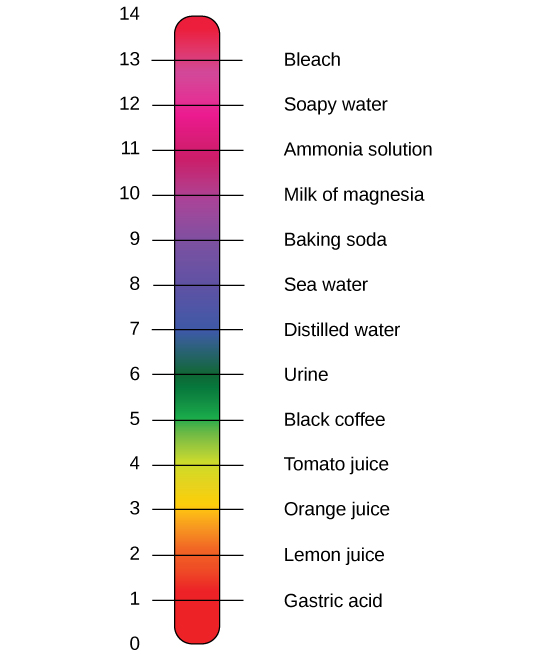The pH scale: The pH scale measures the concentration of hydrogen ions (H+) in a solution.

Non-neutral pH readings result from dissolving acids or bases in water. Using the negative logarithm to generate positive integers, high concentrations of hydrogen ions yield a low pH, and low concentrations a high pH.

An acid is a substance that increases the concentration of hydrogen ions (H+) in a solution, usually by dissociating one of its hydrogen atoms. A base provides either hydroxide ions (OH) or other negatively-charged ions that react with hydrogen ions in solution, thereby reducing the concentration of H+ and raising the pH.

Strong Acids and Strong Bases

The stronger the acid, the more readily it donates H+. For example, hydrochloric acid (HCl) is highly acidic and completely dissociates into hydrogen and chloride ions, whereas the acids in tomato juice or vinegar do not completely dissociate and are considered weak acids; conversely, strong bases readily donate OH and/or react with hydrogen ions. Sodium hydroxide (NaOH) and many household cleaners are highly basic and give up OH rapidly when placed in water; the OHions react with H+ in solution, creating new water molecules and lowering the amount of free H+ in the system, thereby raising the overall pH. An example of a weak basic solution is seawater, which has a pH near 8.0, close enough to neutral that well-adapted marine organisms thrive in this alkaline environment.

Buffers

How can organisms whose bodies require a near-neutral pH ingest acidic and basic substances (a human drinking orange juice, for example) and survive? Buffers are the key. Buffers usually consist of a weak acid and its conjugate base; this enables them to readily absorb excess H+ or OH, keeping the system’s pH within a narrow range.

Maintaining a constant blood pH is critical to a person’s well-being. The buffer that maintains the pH of human blood involves carbonic acid (H2CO3), bicarbonate ion (HCO3), and carbon dioxide (CO2). When bicarbonate ions combine with free hydrogen ions and become carbonic acid, hydrogen ions are removed, moderating pH changes. Similarly, excess carbonic acid can be converted into carbon dioxide gas and exhaled through the lungs; this prevents too many free hydrogen ions from building up in the blood and dangerously reducing its pH; likewise, if too much OH is introduced into the system, carbonic acid will combine with it to create bicarbonate, lowering the pH. Without this buffer system, the body’s pH would fluctuate enough to jeopardize survival.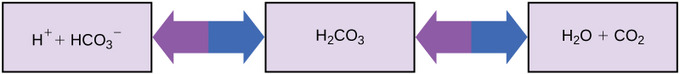Buffers in the body: This diagram shows the body’s buffering of blood pH levels: the blue arrows show the process of raising pH as more CO2 is made; the purple arrows indicate the reverse process, lowering pH as more bicarbonate is created.

Antacids, which combat excess stomach acid, are another example of buffers. Many over-the-counter medications work similarly to blood buffers, often with at least one ion (usually carbonate) capable of absorbing hydrogen and moderating pH, bringing relief to those that suffer “heartburn” from stomach acid after eating.

Brønsted Acids and Bases

A Brønsted acid is any species capable of donating a proton; a Brønsted base is any capable of accepting a proton.

Learning Objectives

Identify the Brønsted acid, Brønsted base, conjugate acid, and conjugate base in an acid-base reaction.

Key Takeaways

Key Points

• The Brønsted-Lowry theory is defined by the following reaction: acid + base <=> conjugate base + conjugate acid. A conjugate base forms after the acid loses a proton, while the conjugate acid forms when the base accepts the proton. The reaction can proceed in either direction.
• The Brønsted-Lowry acid-base theory has several advantages over the Arrhenius theory: for example, only the Brønsted theory describes the reaction between acetic acid and ammonia, which does not produce hydrogen ions in solution.
• Water is amphoteric, which means it can act as either an acid or a base.

Key Terms

• Brønsted-Lowry base: any chemical species that acts as an acceptor of protons
• Brønsted-Lowry acid: any chemical species that acts as a donor of protons
• conjugate base: the species formed after an acid donates its proton; typically a weak base
• conjugate acid: the species formed after a base accepts a proton; typically a weak acid

In chemistry, the Brønsted-Lowry theory is an acid-base theory, independently proposed by Johannes Nicolaus Brønsted and Thomas Martin Lowry in 1923. In this system, acids and bases are defined as follows: an acid is any species that is able to donate a hydrogen cation (H+, a proton); a base is any species with the ability to accept a hydrogen cation (H+). To that end, if a compound is to behave as an acid by donating a proton, there must be a base to accept that proton; the Brønsted-Lowry concept is therefore defined by the reaction:

acid + base ⇌ conjugate base + conjugate acid

The conjugate base is the ion or molecule that remains after the acid has donated its proton, and the conjugate acid is the species created after the base accepts the proton. The reaction can proceed either forward backward; in each case, the acid donates a proton to the base.

The Brønsted-Lowry acid-base theory has several advantages over the Arrhenius theory. Recall that the Arrhenius theory defines an acid as any species that increases the concentration of H+/H3O+ in solution. Consider the following reactions of acetic acid (CH3COOH), the organic acid that gives vinegar its characteristic taste:

1. CH3COOH + H2O ⇌ CH3COO + H3O+
2. CH3COOH + NH3 ⇌ CH3COO + NH4+

Both theories easily describe the first reaction: CH3COOH acts as an Arrhenius acid because it acts as a source of H3O+ when dissolved in water, and it acts as a Brønsted acid by donating a proton to water. In the second example CH3COOH undergoes the same transformation, in this case donating a proton to ammonia (NH3); this cannot be described using the Arrhenius definition of an acid, however, because the reaction does not produce H3O+.

Amphoterism of Water

Water is amphoteric, which means it can act as either an acid or a base. In the reaction between acetic acid, CH3CO2H, and water, H2O, water acts as a base. The acetate ion CH3CO2 is the conjugate base of acetic acid, and the hydronium ion H3O+ is the conjugate acid of the base, water:

CH3COOH + H2O ⇌ CH3COO + H3O+

Water can also act as an acid, as when it reacts with ammonia. The equation given for this reaction is:

H2O + NH3 ⇌ OH + NH4+

Here, H2O donates a proton to NH3. The hydroxide ion is the conjugate base of water, which acts as an acid, and the ammonium ion is the conjugate acid of the base, ammonia.

Acid-Base Titrations

Acid-base titration can determine the concentrations of unknown acid or base solutions.

Learning Objectives

Compute the concentration of an unknown acid or base given its volume and the volume and concentration of the standardized titrant.

Key Takeaways

Key Points

• An acid – base titration is a quantitative analysis of acids and bases; through this process, an acid or base of known concentration neutralizes an acid or base of unknown concentration.
• The titration progress can be monitored by visual indicators, pH electrodes, or both.
• The reaction’s equivalence point is the point at which the titrant has exactly neutralized the acid or base in the unknown analyte; if you know the volume and concentration of the titrant at the equivalence point, you can calculate the concentration of a base or acid in the unknown solution.

Key Terms

• analyte: the unknown solution whose concentration is being determined in the titration
• equivalence point: the point at which an added titrant’s moles are stoichiometrically equal to the moles of acid/base in the sample; the smallest amount of titrant needed to fully neutralize or react with the analyte
• acid-base titration: determines the concentration of an acid or base by exactly neutralizing it with an acid or base of known concentration
• titrant: the standardized (known) solution (either an acid or a base) that is added during titration

Setting up an Acid-Base Titration

An acid-base titration is an experimental procedure used to determined the unknown concentration of an acid or base by precisely neutralizing it with an acid or base of known concentration. This lets us quantitatively analyze the concentration of the unknown solution. Acid-base titrations can also be used to quantify the purity of chemicals.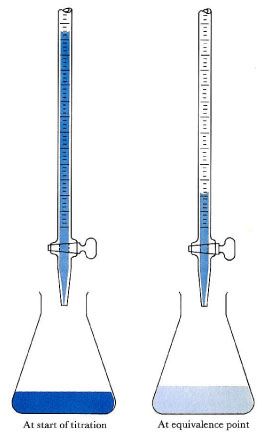Acid-base titration: The solution in the flask contains an unknown number of equivalents of base (or acid). The burette is calibrated to show volume to the nearest 0.001 cm3. It is filled with a solution of strong acid (or base) of known concentration. Small increments are added from the burette until, at the end point, one drop changes the indicator color permanently. (An indication of the approaching equivalence point is that the indicator changes color but changes back after stirring.) At the equivalence point, the total amount of acid (or base) is recorded from the burette readings. The number of equivalents of acid and base must be equal at the equivalence point.

Alkalimetry, or alkimetry, is the specialized analytic use of acid-base titration to determine the concentration of a basic (alkaline) substance; acidimetry, or acidometry, is the same concept applied to an acidic substance.

Materials for a Titration Procedure

• burette
• white tile (used to see a color change in the solution)
• pipette
• pH indicator (the type depends on the reactants )
• titrant (a standard solution of known concentration; a common example is aqueous sodium carbonate)
• analyte, or titrand (the solution of unknown concentration)

Equivalence Point Indicators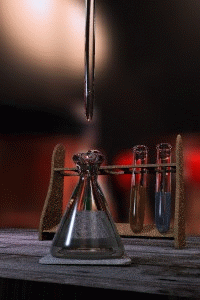Acid-base titration setup: The pink color is caused by the phenolphthalein indicator.

Before you begin the titration, you must choose a suitable pH indicator, preferably one that will experience a color change (known as the “end point”) close to the reaction’s equivalence point; this is the point at which equivalent amounts of the reactants and products have reacted. Below are some common equivalence point indicators:

• strong acid – strong base titration: phenolphthalein indicator
• weak acid – weak base titration: bromthymol blue indicator
• strong acid-weak base titration: methyl orange indicator the base is off the scale (e.g., pH > 13.5) and the acid has pH > 5.5: alizarine yellow indicator
• the base is off the scale (e.g., pH > 13.5) and the acid has pH > 5.5: alizarine yellow indicator
• the base is off the scale (e.g., pH > 13.5) and the acid has pH > 5.5: alizarine yellow indicator
• the acid is off the scale (e.g., pH < 0.5) and the base has pH < 8.5: thymol blue indicator

Estimating the Equivalence Point’s pH

The resulting solution at the equivalence point will have a pH dependent on the acid and base’s relative strengths. You can estimate the equivalence point’s pH using the following rules:

• A strong acid will react with a weak base to form an acidic (pH < 7) solution.
• A strong acid will react with a strong base to form a neutral (pH = 7) solution.
• A weak acid will react with a strong base to form a basic (pH > 7) solution.

When a weak acid reacts with a weak base, the equivalence point solution will be basic if the base is stronger and acidic if the acid is stronger; if both are of equal strength, then the equivalence pH will be neutral. Weak acids are not often titrated against weak bases, however, because the color change is brief and therefore very difficult to observe.

You can determine the pH of a weak acid solution being titrated with a strong base solution at various points; these fall into four different categories: (1) initial pH; (2) pH before the equivalence point; (3) pH at the equivalence point; and (4) pH after the equivalence point.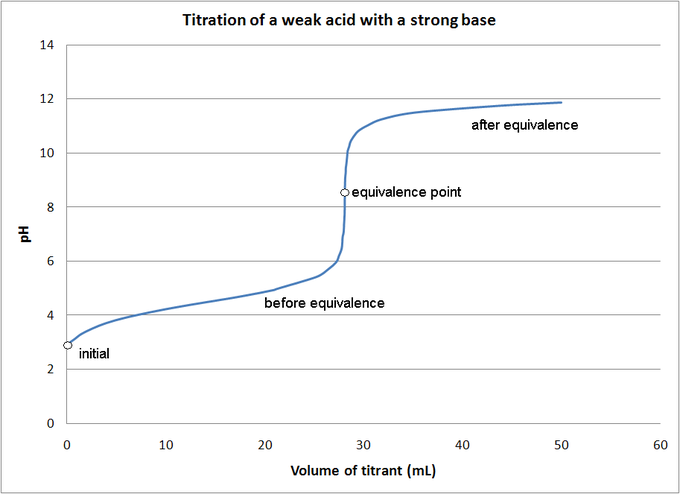Titration of a weak acid by a strong base: The pH of a weak acid solution being titrated with a strong base solution can be found at each indicated point.

Titration Procedure

1. Rinse the burette with the standard solution, the pipette with the unknown solution, and the conical flask with distilled water.
2. Place an accurately measured volume of the analyte into the Erlenmeyer flask using the pipette, along with a few drops of indicator. Place the standardized solution into the burette, and indicate its initial volume in a lab notebook. At this stage, we want a rough estimate of the amount of known solution necessary to neutralize the unknown solution. Let the solution out of the burette until the indicator changes color, and record the value on the burette. This is the first titration and it is not very precise; it should be excluded from any calculations.
3. Perform at least three more titrations, this time more accurately, taking into account where the end point will roughly occur. Record the initial and final readings on the burette, prior to starting the titration and at the end point, respectively. (Subtracting the initial volume from the final volume will yield the amount of titrant used to reach the endpoint.)
4. The end point is reached when the indicator permanently changes color.

Chemistry 12.6b Calculating Titrations – YouTube: This lesson shows how to carry out calculations for titrations and neutralization reactions to find the concentration of an unknown acid or base. It also discusses how to deal with polyprotic acids and bases with multiple hydroxides.

Gas Evolution Reactions

A gas evolution reaction is a chemical process that produces a gas, such as oxygen or carbon dioxide.

Learning Objectives

Identify when a reaction will evolve a gas.

Key Takeaways

Key Points

• Acids react with carbonates to produce a salt, carbon dioxide, and water.
• Limewater can be used to detect the presence of carbon dioxide evolved in a reaction.
• Hydrogen gas and a metal salt are produced when acids react with metals.

Key Terms

• gas evolution reaction: a chemical process that produce a gas, such as oxygen or carbon dioxide
• limewater: an aqueous solution of Ca(OH)2; a common indicator used to detect the presence of carbon dioxide gas

A gas evolution reaction is a chemical process that produces a gas, such as oxygen or carbon dioxide. In the following examples, an acid reacts with a carbonate, producing salt, carbon dioxide, and water, respectively.

Nitric acid reacts with sodium carbonate to form sodium nitrate, carbon dioxide, and water:

$2\text{HNO}_{3}(aq)+\text{Na}_2\text{CO}_{3}(aq)\rightarrow2\text{NaNO}_{3}(aq)+\text{CO}_{2}(g)+\text{H}_{2}\text{O}(l)$

Sulfuric acid reacts with calcium carbonate to form calcium sulfate, carbon dioxide, and water:

$\text{H}_{2}\text{SO}_{4}(aq)+\text{CaCO}_{3}(aq)\rightarrow\text{CaSO}_{4}(aq)+\text{CO}_{2}(g)+\text{H}_{2}\text{O}(l)$

Hydrochloric acid reacts with calcium carbonate to form calcium chloride, carbon dioxide, and water:

$2\text{HCl}(aq)+\text{CaCO}_{3}(aq)\rightarrow\text{CaCl}_{2}(aq)+\text{CO}_{2}(g)+\text{H}_{2}\text{O}(l)$

The following setup demonstrates this type of reaction: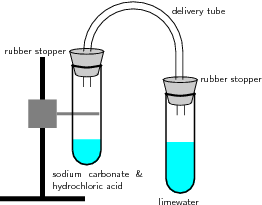Reaction of acids with carbonates: In this reaction setup, lime water is poured into one of the test tubes and sealed with a stopper. A small amount of hydrochloric acid is carefully poured into the remaining test tube. A small amount of sodium carbonate is added to the acid, and the tube is sealed with a rubber stopper. The two tubes are connected. As a result of the acid-carbonate reaction, carbon dioxide is produced and the lime water turns milky.

The test tube on the right contains limewater (a solution of calcium hydroxide, Ca(OH)2). On the left, a solution of hydrochloric acid has been added to a solution of sodium carbonate to generate $\text{CO}_2(g)$. The test tubes are sealed with rubber stoppers and connected with a delivery tube. As the reaction proceeds, the limewater on the right turns from clear to milky; this is due to the $\text{CO}_2(g)$ reacting with the aqueous calcium hydroxide to form calcium carbonate, which is only slightly soluble in water. The entire experiment is illustrated in the following video:

Science Experiment: Carbon Dioxide (CO) & Limewater (Chemical Reaction): Carbon dioxide (formed from bicarbonate) and an acid react with limewater, turning it from clear to milky.

When this experiment is repeated with nitric or sulfuric acid instead of HCl, it yields the same results: the clear limewater turns milky, indicating the production of carbon dioxide.

Oxidation of Metals in Acidic Solution

The oxidation of a metal in acidic solution will yield a metal salt and hydrogen gas:

$2\text{HCl}_{\left(aq\right)}+\text{Zn}_{\left(s\right)}\rightarrow\text{ZnCl}_{2\left(aq\right)}+\text{H}_{2\left(g\right)}$

Acid and metal reaction: Hydrochloric acid oxidizes zinc to produce an aqueous metal salt and hydrogen gas bubbles.

Recall that oxidation refers to a loss of electrons, and reduction refers to the gain of electrons. In the above redox reaction, neutral zinc is oxidized to Zn2+, and the acid, H+, is reduced to H2(g). The oxidation of metals by strong acids is another common example of a gas evolution reaction.